DIY

# How to make a water level sensor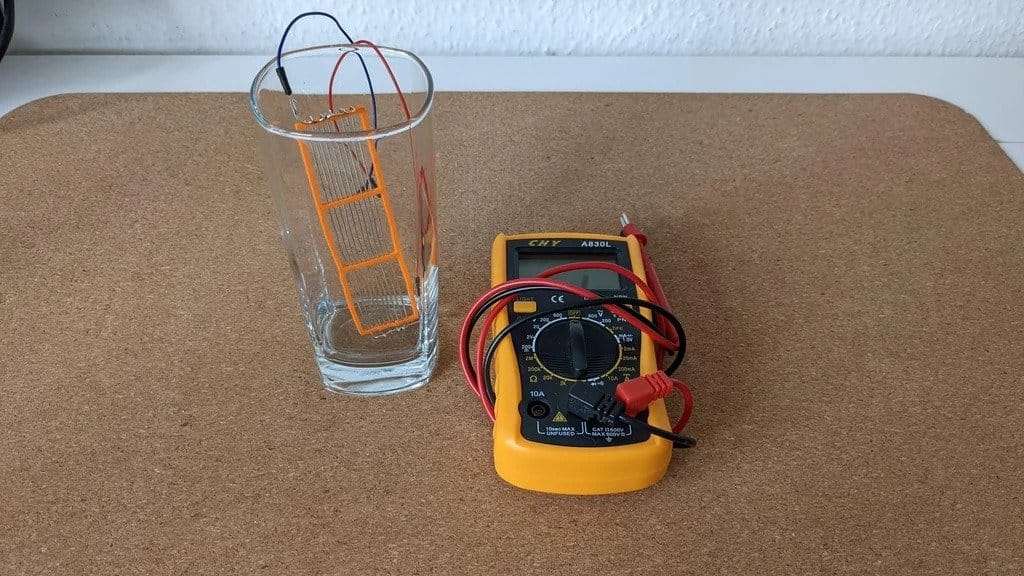In this article, the wizard will tell us how you can make your own water level sensor. This sensor is an analogue of the sensor for Arduino and also works with this board.
Tools and materials: -3D-printer; -Arduino uno;
-Wire 0.8 mm;
-Connecting wires;
-Resistors; -Soldering iron; -Multimeter; -Computer;
-Cable for connecting to Arduino.
Step one: the principle of the sensor
First, we need to understand how the sensor works.
There are several parallel copper traces on the board. They alternately connect to the VCC and GND of the Arduino. While the sensor is dry, there is no electrical connection between them, but as the water level rises, they do. You can think of them as a variable resistor. The higher the water level rises, the lower the resistance falls.
Resistance can be measured with a voltage divider as shown in the figure below. This is a simple circuit in which R1 is a fixed resistor and R2 is a variable resistor. Since the current through all resistors in a row is the same, the voltage drop across each resistor depends on its resistance — or a fraction of the total resistance in the circuit. Thus, when the water level increases, the voltage measured at the analog input increases until the entire sensor is covered with water.
If you know the total height of the sensor and the maximum and minimum resistance values ​​at the inlet, you can calculate the water level .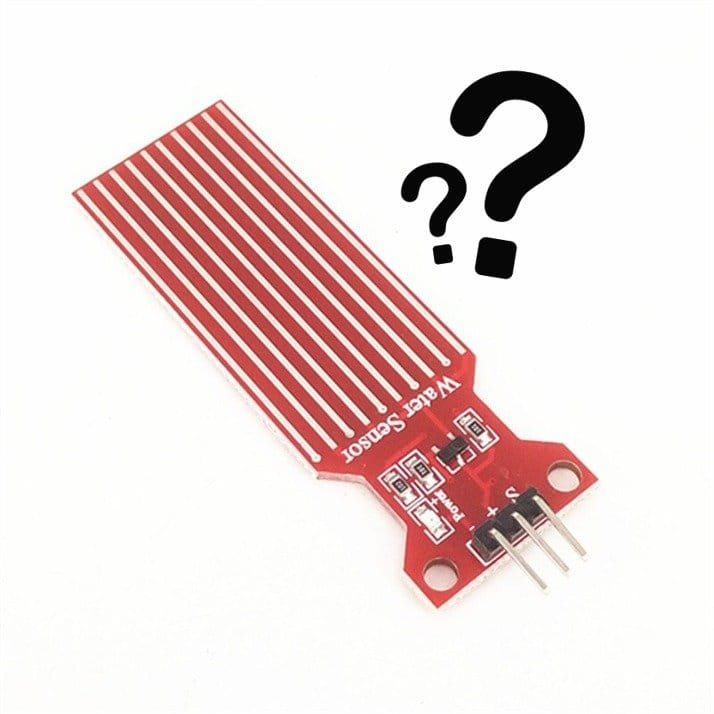Step two: making a sensor
To make a sensor, you need to print a frame on a 3D printer. Frame length 10 cm.
Then you need to pull the wire through the holes on the side, as shown in the photo, cut it a couple of centimeters longer than the frame on both sides and repeat the process for all other holes.
At the end you need to bend the wire and solder the ends together. Then solder one jumper cable from each end.
WaterLevelSensor_Frame.stl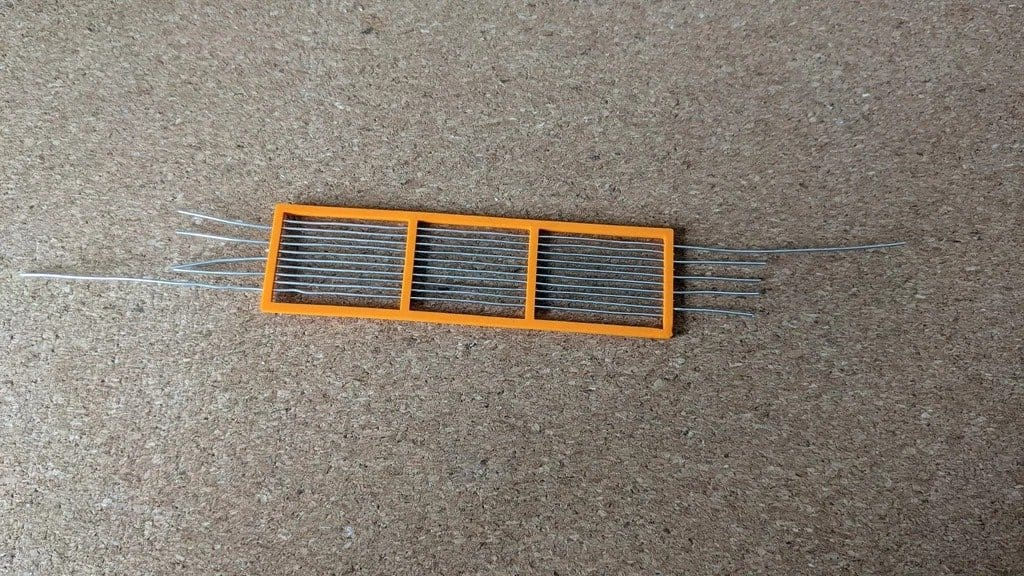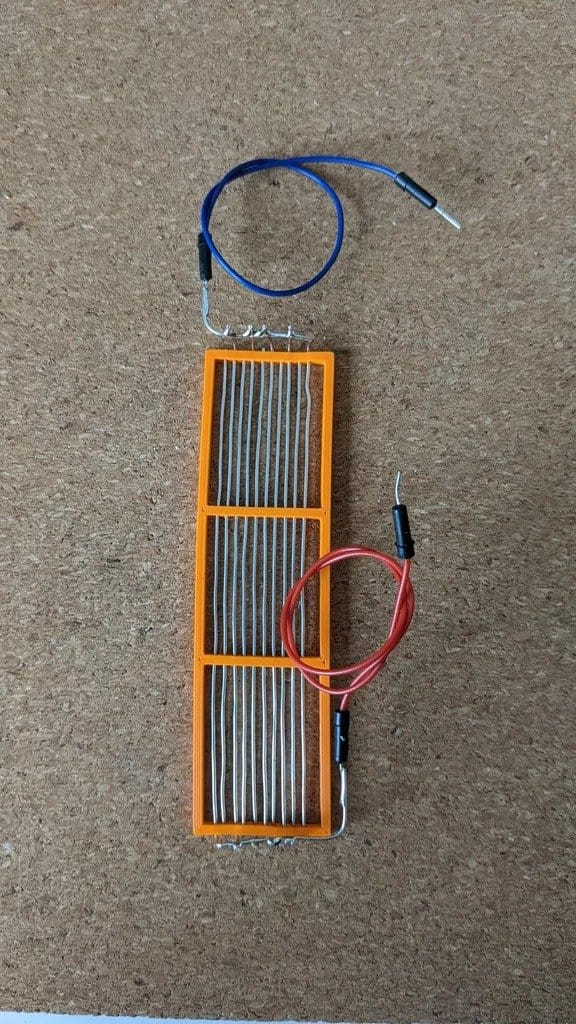Step three: resistance measurement
Now you need to measure the resistance of the sensor with a multimeter, while it is still dry.
Depending on this value, you need to choose a resistor – its value should be somewhere in the same range as that of the sensor.Step four: schematic and code
The master assembles a schematic on a breadboard. Due to the fact that there was no water level sensor in the editor, a photoresistor was designated instead.
After installing the circuit, you need to upload the code to Arduino.
First you need to uncomment line 25 'Serial.println (sensorVal). Next, open the Serial Monitor (in the upper right corner of the Arduino IDE) and see what values ​​there will be. The analog input of the Arduino accepts voltages from 0 to 5 V and converts them to values ​​between 0 and 1024. Since the homemade sensor is connected directly to the Arduino and has no circuitry to stabilize the values, they will be quite unstable.
The upper limit should be approximately equal to the value which can be read on a serial monitor. In this case, it is somewhere around 550. You need to change the value of the upperLimit variable to this value.
Then we completely immerse the sensor in water and see how the serial output changes. Change the variable lowerLimit to this value.
To get an accurate reading, you need to have a large difference between the upper and lower limits. If they are close to each other, even a small change in value due to instability will greatly change the reading. It is best if the upper and lower limits are several hundred digits apart.
To increase the difference, you can try different resistor values. The lower its rating, the higher the sensor values ​​should be.
Now you can comment out line 25 again to see only the measurement result.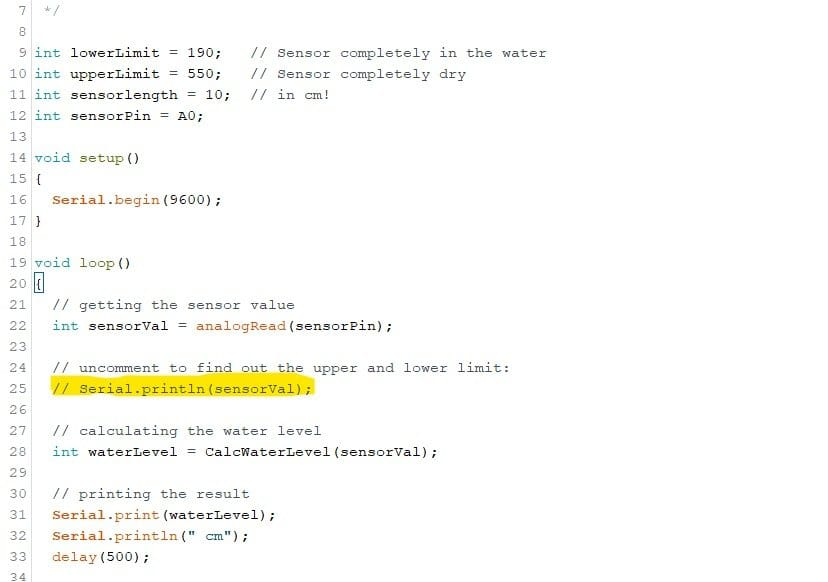Step five: testing